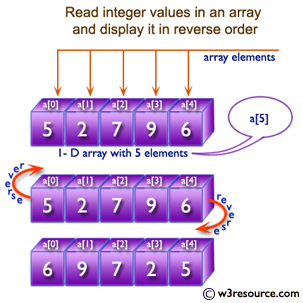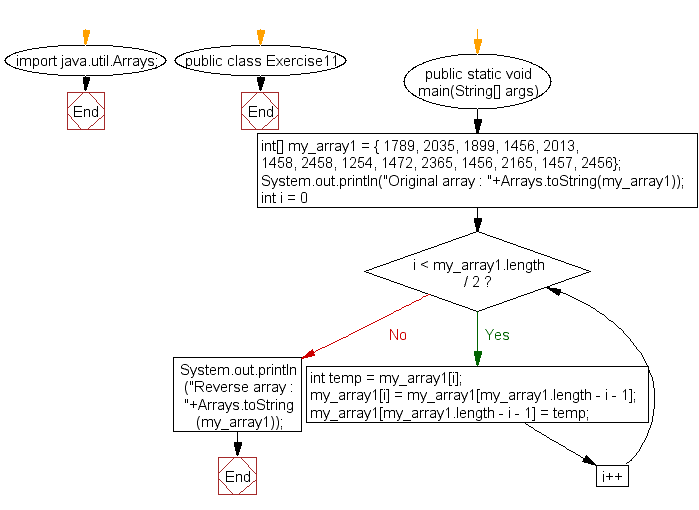﻿ Java exercises: Reverse an array of integer values - w3resource# Java Array Exercises: Reverse an array of integer values

## Java Array: Exercise-11 with Solution

Write a Java program to reverse an array of integer values.

Pictorial Presentation:Sample Solution:

Java Code:

``````import java.util.Arrays;
public class Exercise11 {
public static void main(String[] args){
int[] my_array1 = {
1789, 2035, 1899, 1456, 2013,
1458, 2458, 1254, 1472, 2365,
1456, 2165, 1457, 2456};
System.out.println("Original array : "+Arrays.toString(my_array1));
for(int i = 0; i < my_array1.length / 2; i++)
{
int temp = my_array1[i];
my_array1[i] = my_array1[my_array1.length - i - 1];
my_array1[my_array1.length - i - 1] = temp;
}
System.out.println("Reverse array : "+Arrays.toString(my_array1));
}
}
```
```

Sample Output:

```Original array : [1789, 2035, 1899, 1456, 2013, 1458, 2458, 1254, 1472, 2365, 1456, 2165, 1457, 2456]
Reverse array : [2456, 1457, 2165, 1456, 2365, 1472, 1254, 2458, 1458, 2013, 1456, 1899, 2035, 1789]
```

Flowchart:Visualize Java code execution (Python Tutor):

Java Code Editor:

Improve this sample solution and post your code through Disqus

What is the difficulty level of this exercise?

Test your Programming skills with w3resource's quiz.

﻿

## Java: Tips of the Day

countOccurrences

Counts the occurrences of a value in an array.

Use Arrays.stream().filter().count() to count total number of values that equals the specified value.

```public static long countOccurrences(int[] numbers, int value) {
return Arrays.stream(numbers)
.filter(number -> number == value)
.count();
}
```

Ref: https://bit.ly/3kCAgLb